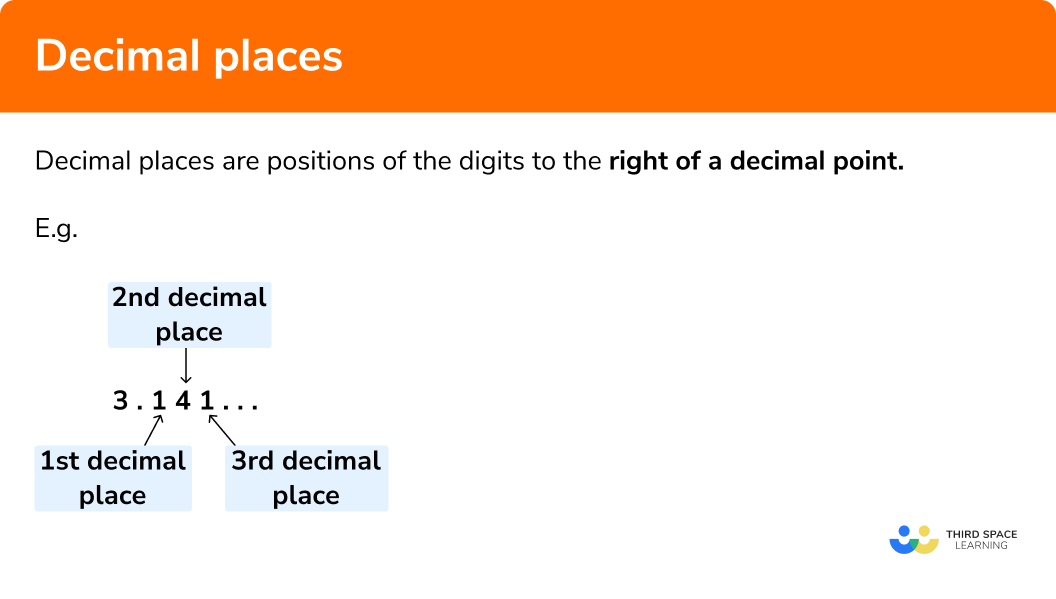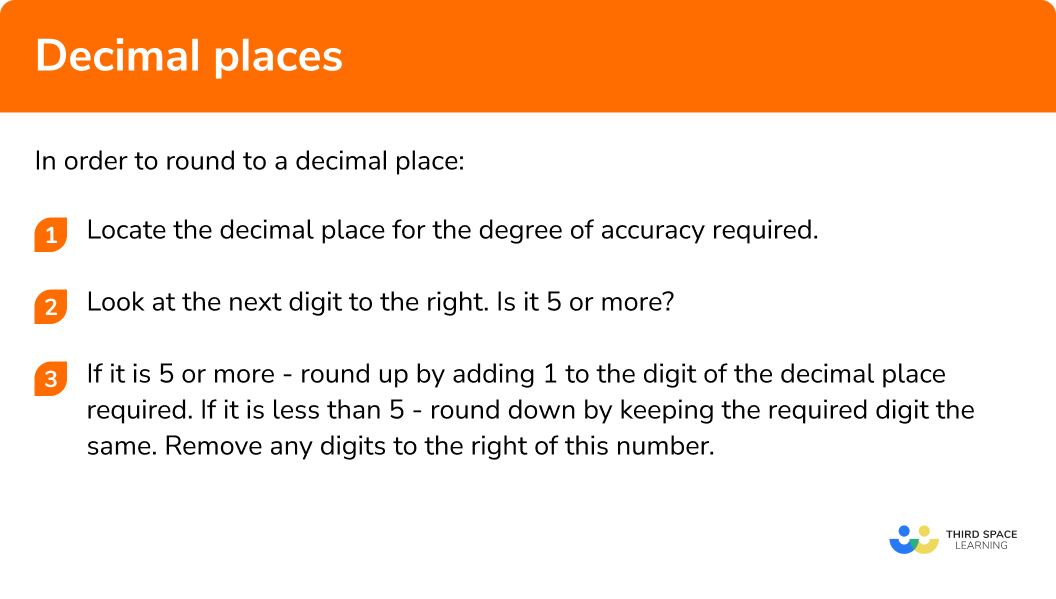# Decimal Places

Here we will learn about decimal places including how to round numbers to one decimal place, two decimal places and three decimal places.

There are also rounding to decimal places worksheets based on Edexcel, AQA and OCR exam questions, along with further guidance on where to go next if you’re still stuck.

## What are decimal places?

Decimal places are positions of the digits to the right of a decimal point.

Rounding numbers to decimal places is shortening a decimal number to a given degree of accuracy. To do this we find the decimal place we wish to round to and look at the digit to the right of that number.
If the number after the chosen accuracy is a 5 or more than 5, we round up.
If it is less than 5, we round down.

It is important to give the number of digits required after the decimal point, even if the last digit would be a zero.

E.g.
Round 27.6273 to 2 decimal places (2.d.p)

### What is rounding to a decimal place?## How to round to decimal places

In order to round to a decimal place:

1. Locate the decimal place for the degree of accuracy required.
2. Look at the next digit to the right. Is it 5 or more?
3. If it is 5 or more – round up by adding 1 to the digit of the decimal place required.
If it is less than 5 – round down by keeping the number of the required digit the same. Remove any digits to the right of this number.

### Explain how to round to a decimal place in 3 steps## Rounding to decimal place examples

### Example 1: rounding to one decimal place

Round 36.248 to the nearest tenth.

1. Locate the decimal for the degree of accuracy required.

The digit representing tenths is the first number to the right of the decimal point.

2Look at the digit to the right. Is it 5 or more?

36.2¦48

4 is less than 5.

3If it is 5 or more – round up by adding 1 to the digit of the decimal place required. If it is less than 5 – round down by keeping the required digit the same and removing all of the digits to the right.

As 4 is less than 5, we round down.

36.248 is 36.2 to the nearest tenth.

### Example 2: rounding to two decimal places

A calculator displayed an answer to a calculation as 53.2983613. What is this given to two decimal places?

Locate the decimal for the degree of accuracy required.
We need the second digit after the decimal point.

Look at the digit to the right. Is it 5 or more?

If it is 5 or more – round up by adding 1 to the digit of the decimal place required. If it is less than 5 – round down by keeping the required digit the same and removing all of the digits to the right.

### Example 3: rounding to three decimal places

Round 0.071562 to three decimal places.

Locate the decimal for the degree of accuracy required.
We need the third digit after the decimal point.

Look at the digit to the right. Is it 5 or more?

If it is 5 or more – round up by adding 1 to the digit of the decimal place required. If it is less than 5 – round down by keeping the required digit the same and removing all of the digits to the right.

### Common misconceptions

• Adding 1 to all of the previous digits when rounding up

A common error when rounding up is to increase all of the digits to the left by 1.
E.g.
If 53.683 was rounded to one decimal place, a student may write 64.7 instead of the correct answer 53.7.

• Leaving out zeros when rounding a 9 up

It is important to remember to write the number of decimals the degree of accuracy requires. If 23.997 is rounded to two decimal places it must be given as 24.00.

Decimal places are part of our series of lessons to support revision on rounding numbers. You may find it helpful to start with the main rounding numbers lesson for a summary of what to expect, or use the step by step guides below for further detail on individual topics. Other lessons in this series include:

### Practice decimal places questions

1. Round 5.162 to one decimal place.

5.15.26.25.0The first decimal place is 1 . The value to the right of this is 6 , which is greater than 5, so round up. The answer is 5.2 .

2. Round 36.715 to the nearest tenth.

4036.836.737.7The tenth digit is 7 . The value to the right of this is 1 , which is less than 5 , so round down. The answer is 36.7 .

3. Round 21.7349 to the nearest hundredth.

21.7421.7321.8321.80The hundredth digit is 3 . The value to the right of this is 4 , which is less than 5 , so round down. The answer is 21.73 .

4. Round 1.73682 to two decimal places.

1.700.741.741.84The second digit after the decimal point is 3 . The value to the right of this is 6 , which is greater than 5 , so round up. The answer is 1.74 .

5. Round 0.69987 to three decimal places.

0.7000.6990.70.600The third digit after the decimal point is 9 and the value to the right of this is 8 .
8 is greater than 5 so round up. Increasing 9 by 1 gives us 10 , so we need to increase the previous 9 by 1 which also gives us 10 . Therefore we need to increase the 6 by 1 to give us 7 .We need to include the zeros in our answer so that it is written to 3d.p. The answer is 0.700 .

6. Round 0.03197 to the nearest thousandth.

0.0310.0400.1420.032The thousandth digit is 1 . The value to the right of this is 9 , which is greater than 5 , so round up. The answer is 0.032 .

### Decimal places GCSE questions

1.(a) Write 27.932 correct to two decimal places.

(b) Write 3.1415927 correct to three decimal places.

(c) Write 286.976 correct to one decimal place.

(3 marks)

(a) 27.93

(1)

(b) 3.142

(1)

(c) 287.0

(1)

2. Ben was subtracting some decimals on his calculator and the display showed the answer as 7.8469 . Ben needed to round his answer to two decimal places. He wrote his answer is 7.84 . Explain the mistake Ben has made in his rounding, and write the correct answer.

(2 marks)

Ben rounded down when he should have rounded up.

(1)

(1)

3. (a) Use a calculator to work out \frac{4.6\times3.7}{\sqrt6.2}. write down all of the digits on your calculator screen.

(2 marks)

(1)

Rounded correctly to 6.84

(1)

## Learning checklist

You have now learned how to:

• Apply and interpret limits of accuracy when rounding

## Still stuck?

Prepare your KS4 students for maths GCSEs success with Third Space Learning. Weekly online one to one GCSE maths revision lessons delivered by expert maths tutors.

Find out more about our GCSE maths tuition programme.